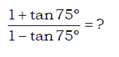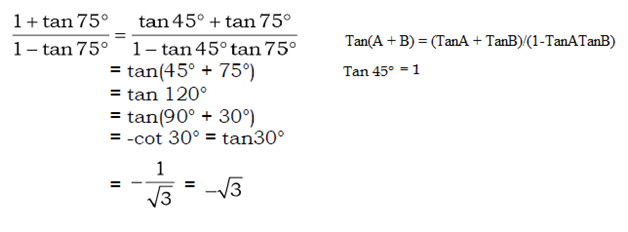# SSC CGL 2018 Practice Test Papers | Quantitative Aptitude (Day-42)

Dear Aspirants, Here we have given the Important SSC CGL Exam 2018 Practice Test Papers. Candidates those who are preparing for SSC CGL 2018 can practice these questions to get more confidence to Crack SSC CGL 2018 Examination.

[WpProQuiz 3380]

Click “Start Quiz” to attend these Questions and view Explanation

1)a) 2/√3

b) √3

c) -√3

d) 1/√3

2) A profit of Rs 1200 is made by selling an article if one-third of it is sold at 9% profit and the remaining at 3% loss. What is the cost price of the article?

a) 100000

b) 120000

c) 150000

d) 200000

3) 45÷9×√2025=?

a) 500

b) 435

c) 225

d) 495

4) LCM of two co prime numbers is 475. What is the product of two numbers?

a) 1905

b) 475

c) 2255

d) Cannot be determined

5) Two trains start at the same time from A to B and proceed towards B to A having speeds of 38 km/hr and 32 km/hr respectively.When they meet, it is found that one train has moved 54 km more than the other. The distance between A and B is –

a) 624 km

b) 630 km

c) 628 km

d) 625 km

6) A and B together can complete a work in 5 days. They start working together but, after 3 days B left the work. If the work is completed after 3 more days, B alone could do the work in –

a) 12 days

b) 15 days

c) 13 days

d) 14 days

7) There is a ratio of 8 : 9 between two numbers. If 30 percent of the first is 36, then 60 % of the second number is –

a) 45

b) 72

c) 81

d) 90

8) A loss of 18 % gets converted into a profit of 15 % when the selling price is increased by Rs. 198. The cost price of the article is –

a) Rs. 450

b) Rs. 575

c) Rs. 500

d) Rs. 600

9) A shopkeeper marked a product 75% above cost price and sold it after two consecutive discounts of x% and 20%. If the shopkeeper made a profit of 26% then find the value of x.

a) 10

b) 20

c) 30

d) 25

10) Find the volume of a cone whose radius and slant height is 7 cm and 25 cm, respectively.

a) 1028 cm3
b) 1436 cm3
c) 1232 cm3
d) None of theseIt can be calculated as:

CP = 1200 *100 / [1/3 *9 + 2/3 * (-3)] = 1,20,000

(45÷9)×√2025 = 5×45 = 225

HCF of co prime numbers = 1

Product of two numbers= LCM x HCF

= 475×1

So, the product = 475

Speed of A, S1=38 kmph

Speed of B, S2= 32 kmph

Relative speed= (38+32) = 70 kmph

D1 = x+54

D2 = x

D1 = x+54 = 38 T

X = 38T – 54 —– (i)

D2 = x = S2T

X=32T —– (ii)

From (i) and (ii)

Time (t) = 9h

Distance between two stations = 38T+32T = 70T

=70×9 = 630 km

A+B=1/5

Work Completed by A and B in three days =3/5

Remaining work =2/5

2/5——-3 days

1———?

?=15/2 days

A can do the work in 15/2 days

One day work of B= 1/5 – 2/15

=1/15 days

B can do the work in 15 days

Let the first number and Second number be 8x and 9x respectively.

30/100×8x=36

X=15

60/100×9x=60/100×9×15 = 81

(18% + 15%) of CP=198

33% CP=198

100% CP=198/33×100 = 600

Let CP = 100

MP = 175

175×(100 – x)/100×80/100 = 126

100 – x = 90

X = 10

Volume of Cone= 1/3 πr2h

L=25, r=7

l2=h2+r2

h2 = l2 – r2

= 252 – 72

=576

H = 24

V= 1/3 × 22/7 × 7 × 7×24 = 1232 cm3